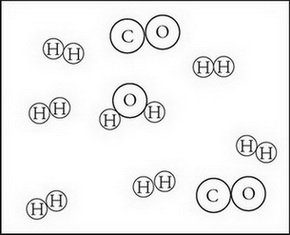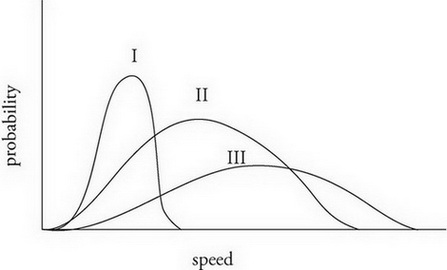# AP Chemistry Practice Test 8

### Test Information13 questions19 minutes

`Questions 1-5 refer to the following information.The enthalpy values for several reactions are as follows:(I)   CH4(g) + H2(g) → C(s) + H2O(g) &DELTA;H = -131 kJ/molrxn(II)   CH4(g) + H2O(g) → 3H2(g) + CO(g) &DELTA;H = 206 kJ/molrxn(III)   CO(g) + H2O(g) → CO2(g) + H2(g)&DELTA;H = - 41 kJ/molrxn(IV)   CH4(g) + 2O2(g) → CO2(g) + H2O(l) &DELTA;H = -890 kJ/molrxn`

1. In which of the reactions does the amount of energy released by the formation of bonds in the products exceed the amount of energy necessary to break the bonds of the reactants by the greatest amount?

2. In which of the reactions is the value for &DELTA;S the most positive?

3. Regarding reaction I, how would the addition of a catalyst affect the enthalpy and entropy changes for this reaction?

Enthalpy Entropy

4.Regarding reaction II, to achieve the products present in the above diagram how many moles of each reactant must be present prior to the reaction?

5. Regarding reaction IV, how much heat is absorbed or released when 2.0 mol of CH4(g) reacts with 2.0 mol of O2(g) ?

6. London dispersion forces are caused by

7. What is the general relationship between temperature and entropy for diatomic gases?

8. Which of the following pairs of ions would make the best buffer with a basic pH?

Ka for HC3H2O2 = 1.75 × 10-5

Ka for HPO42- = 4.8 × 10-13

9. A solution contains a mixture of four different compounds: KCl(aq), Fe(NO3)3(aq), MgSO4(aq), and N2H4(aq). Which of these compounds would be easiest to separate via distillation?

10.Identify the three gases represented on the Maxwell-Boltzmann diagram above. Assume all gases are at the same temperature.

I    II    III

11. A sample of solid MgCl2 would be most soluble in which of the following solutions?

12. Most transition metals share a common oxidation state of +2. Which of the following best explains why?

13. 2Ag+(aq) + Fe(s) → 2Ag(s) + Fe2+(aq)

Which of the following would cause an increase in potential in the voltaic cell described by the above reaction?